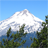# How to convert a line to polygon in JS API 4.x

1410
1
12-19-2019 06:31 PMby
New Contributor IIIHi,

I couldn't seem to find anywhere in the JavaScript API 4.x that can construct a polygon geometry from a set of polyline geometries. Can someone point me to the right method?

Thanks.

Tags (2)
1 Solution

Accepted SolutionsNew Contributor III

As far as the line geometries are connected, each line geometry first coordinate and last coordinate are identical.  Polygon  geometries can be constructed by passing the coordiantes from Polyline geometry into Polygon Geometry as below.

From your post, I assume you are thinking of solution specifically for intersection lines into multiple geometries?

In that case it is two step process, convert the grey lines into single polygon geometry and apply the intersection function to generate the polygons.

var polyline = {
type: "polyline"// autocasts as new Polyline()
paths: [
[-111.352.68],
[-9849.5],
[-93.9429.89],
[-111.352.68]
]
};
var polygon = {
type: "polygon"// autocasts as new Polygon()
rings: polyline.paths
};New Contributor III

As far as the line geometries are connected, each line geometry first coordinate and last coordinate are identical.  Polygon  geometries can be constructed by passing the coordiantes from Polyline geometry into Polygon Geometry as below.

From your post, I assume you are thinking of solution specifically for intersection lines into multiple geometries?

In that case it is two step process, convert the grey lines into single polygon geometry and apply the intersection function to generate the polygons.

var polyline = {
type: "polyline"// autocasts as new Polyline()
paths: [
[-111.352.68],
[-9849.5],
[-93.9429.89],
[-111.352.68]
]
};
var polygon = {
type: "polygon"// autocasts as new Polygon()
rings: polyline.paths
};